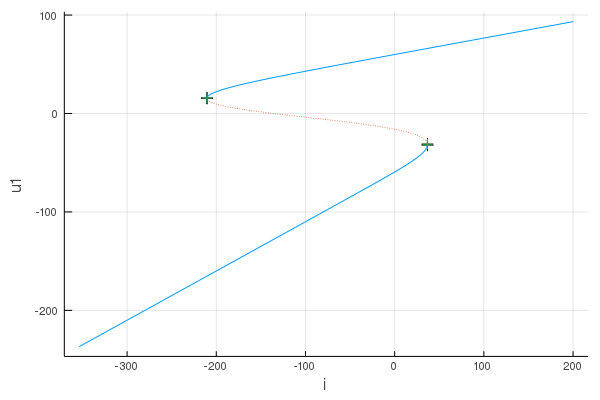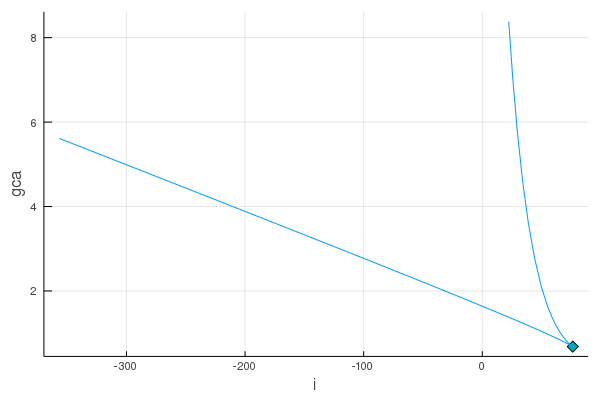Calcium channel model

# Calcium channel model

Calcium channel model taken from PyDSTool example. See:

We define the model parameter using a `NamedTuple`:

``````calcium_param = (
vl = -60,
vca = 120,
i = -220.0,
gl = 2,
gca = 4,
c = 20,
v1 = -1.2,
v2 = 18,
)``````

Define the model as in DifferentialEquations.jl:

``````using Parameters: @unpack

function f(u, p, t)
@unpack vl, vca, i, gl, gca, c, v1, v2 = p
v = u
w = u
dv = (i + gl * (vl - v) - gca * 0.5 * (1 + tanh((v-v1)/v2)) * (v-vca)) / c
dw = v-w
return SVector(dv, dw)
end``````
``f (generic function with 1 method)``

Create an `ODEProblem`:

``````using DiffEqBase: ODEProblem
using StaticArrays: SVector

u0 = SVector(-170.0, -170.0)
tspan = (0.0, 30.0)  # ignored by Bifurcations.jl
ode = ODEProblem(f, u0, tspan, calcium_param)``````
``````[36mODEProblem[0m with uType [36mStaticArrays.SArray{Tuple{2},Float64,1,2}[0m and tType [36mFloat64[0m. In-place: [36mfalse[0m
timespan: (0.0, 30.0)
u0: [-170.0, -170.0]``````

Create a bifurcation problem:

``````using Bifurcations: BifurcationProblem
using Setfield: @lens

param_axis = @lens _.i
prob = BifurcationProblem(ode, param_axis, (-300.0, 100.0))``````

Solve it:

``````using DiffEqBase: init, solve!

solver = init(prob)
solve!(solver)
sol = solver.sol``````
``````Codim1Solution <Continuous>
# sweeps             : 2
# points             : 106
# branches           : 0
``````

Plot it:

``````using Plots

plt = plot(sol)``````Find the left Saddle-Node bifurcation point:

``````using Bifurcations: special_intervals

point_list = sort!(special_intervals(solver), by=p->p.u0[end])
point = point_list``````
``````SpecialPointInterval <Continuous saddle_node>
happened between:
u0 = [15.36, 15.36, -210.476]
u1 = [15.822, 15.822, -210.44]
``````

Numerical continuation of the Saddle-Node bifurcation point:

``````sn_prob = BifurcationProblem(
point,
solver,
(@lens _.gca),
(0.0, 8.0),
)
sn_solver = init(sn_prob)
solve!(sn_solver)``````
``````Codim2Solver <Continuous>
# sweeps             : 2
# points             : 68
# branches           : 0
# cusp               : 1
``````

Plot the phase diagram:

``plt2 = plot(sn_solver.sol)``